# Abstract

We evaluate nucleon and delta sigma-terms and obtain the results 43 MeV < sigmaN < 49 MeV and 28 MeV < <FONT FACE=Symbol>sD</FONT> < 32 MeV, depending on the coupling constants used, which are compatible with values extracted from experiment and produced by other groups. We show that the decay <FONT FACE=Symbol>D ® p</FONT>N explains the relation <FONT FACE=Symbol>sD</FONT> < sigmaN.

Nucleon and delta sigma terms; Delta decay; Effective theories

Nucleon and delta sigma-terms

I. P. CavalcanteI; M. R. RobilottaII; J. Sá BorgesIII; D. de O. SantosI; G. R. S. ZarnauskasII

IDepto. de Física, CCET, Universidade Federal de Mato Grosso do Sul, C.P. 549, C.E.P. 79070-900, Campo Grande, MS, Brazil

IIInstituto de Física, Universidade de São Paulo, C.P. 66318, 05315-970, São Paulo, SP, Brazil

IIIUniversidade do Estado do Rio de Janeiro, Instituto de Física, Rua São Francisco Xavier, 524, Maracanã, Rio de Janeiro, RJ, Brazil

ABSTRACT

We evaluate nucleon and delta sigma-terms and obtain the results 43 MeV < sN< 49 MeV and 28 MeV < sD < 32 MeV, depending on the coupling constants used, which are compatible with values extracted from experiment and produced by other groups. We show that the decay D ® pN explains the relation sD < sN.

Keywords: Nucleon and delta sigma terms; Delta decay; Effective theories

I. INTRODUCTION

QCD is nowadays the main theoretical framework to describe hadronic interactions, but its non-Abelian character makes calculations in the low-energy regime unfeasible. In order to overcome this problem, one may work with either lattice QCD or effective field theories.

Effective theories are constructed by writing the most general Lagrangian containing all the terms allowed by the symmetries of the main theory. In the case of chiral symmetry, the various terms are organized considering the number of pion masses or derivatives of the pion field. This procedure gives meaning to the idea of chiral perturbation theory (ChPT). Considering only u and d quarks, we assume that the effective theory possesses approximate SU(2) × SU(2) chiral symmetry, broken by the small pion mass in the effective theory. An important aspect of chiral dynamics concerns the effects of this breaking of the symmetry over the nucleon and delta resonance masses. The answer to this question is related to sigma-terms.

The delta (D) plays a very important role in low-energy pion-nucleon (pN) scattering and correlated processes, such as the nucleon-nucleon interaction. When the delta is present, the scale of some amplitude denominators is given by the quantity wD ~ M - m. Delta contributions are given by ratios of small quantities and may turn out to be large. In such cases, numerical values adopted for M - m do influence predictions produced by effective theories, especially those that rely on the small scale expansion  or the heavy baryon approximation .

In ChPT, there is a clear conceptual distinction between the bare baryon masses, present in the Lagrangian, and their respective observed values, which include loop corrections. The former should, in principle, be preferred as inputs in the evaluation of theoretical amplitudes. Nevertheless, as there is little information available concerning the bare delta mass, one tends to use physical values in calculations. In most cases, it is reasonable to expect that this would have little numerical importance. On the other hand, in the case of the parameter øD, which is a small quantity, the influence of loop corrections may become relatively large.

According to the Feynman-Hellmann theorem  the mass mB of a baryon B is related to its sigma-term sB by sB = µ2d mB/ dµ2. Therefore the sigma-term provides a measure of the shift in the baryon mass due to chiral symmetry breaking. Whenever it is possible to evaluate sB as a function of µ, the bare masscan be extracted from the relationAs the leading term in sB is proportional to µ2, the difference mB-sB provides a crude estimate for. In the case of the nucleon, one has sN=45 MeV . In ChPT, the leading contribution to sN cannot be predicted theoretically. Formally, it is associated with the constant c1 of the second order Lagrangian [5, 6], which can be extracted from empirical subthreshold information. The situation of the delta is much worse, for pD scattering data are not available. One is then forced to resort to models in order to calculate the delta sigma-term, which is associated with the parameter a1 defined in ref. .

This paper is organized as follows. In section II we review our evaluation of the nucleon and delta sigma-terms, presented in  (the reader is referred to this paper for a detailed description of the work). In section III we show that the relation sD < sN is associated with the fact that the delta can decay. A brief summary is provided in section IV.

II. NUCLEON AND DELTA SIGMA-TERMS

Th nucleon sigma-term obtained by applying ChPT at O(q4) depends on the low-energy constants (LEC's) c1, c2 and c3. In this work we review a model presented in [7, 8].

Data on pN subthreshold coefficients indicate that c2 and c3 are larger than c1 and that their values are approximately saturated by D intermediate states . Thus, up to O(q4), the function sN(t) can be well represented by the leading tree contribution associated with c1, supplemented by the two triangle diagrams shown in Fig.1, involving N and D intermediate states.

The nucleon scalar form factor in momentum space is defined bywhere Lsb is the symmetry breaking term and t = (p¢-p)2. In terms of the quark degrees of freedom, one has Lsb = -(u +d), with= (mu + md)/2. The configuration space scalar form factor is denoted by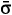N and given bywith q = (p¢-p), in the Breit frame. The nucleon sigma-term, sN, is given by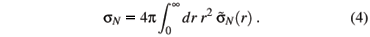The contributions from the diagrams of Fig.1 toN(r) readwhere(r) and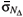(r) denote contributions from diagrams with nucleon and delta as intermediate states, respectively.

In Fig.2, the condensed (QCD vacuum) and empty-space phases are represented by the horizontal lines at values 0 and 1, respectively, whereas the curve sN represents the influence of the nucleon over the condensate. At a critical point R around 0.6 fm, this curve intersects the horizontal line at 1, indicating the possibility of a phase transition. We assume that this phase transition does take place at this point and that the condensate no longer exists in the region r < R.

For this reason, in our previous evaluation of sN [7, 8], we used the expressioninstead of eq. (4). This procedure is the basis of our model and is more extensively discussed in ref. .

In the numerical determination of sN, we consider three possibilities for the pND coupling constant. The corresponding results, given in table 1, are quite close to the value extracted from experiment by Gasser, Leutwyler and Sainio , namely, sN = 45 MeV.

The delta scalar form factor is defined as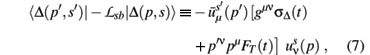whereis the D spinor  and sD and FT are, respectively, the scalar and tensor form factors. The minus sign on the r.h.s. is associated with the conventions used in the free D Lagrangian as in ref. . We assume that the scalar form factor is determined by a short range contact interaction and two long range two-pion processes as in the case of the nucleon.

The values of the distance R for which

D(R)/µ2 = 1 and the values of the delta sigma-term are given in table II, for different choices of the coupling constants gpND and gpDD. Results for the real component of sD are sensitive to the coupling constant gpDD and consistent with that given in ref. , namely sD = (32 ± 3) MeV. On the other hand, our prediction is larger than that quoted in ref. .

The structure of partial contributions for SU(4) coupling constants, namely, gpND = 1.33 and gpDD = 0.75. is given in table III, where core and cloud refer to regions inside and outside the cutting radius R, respectively.

III. LOOP INTEGRALS

The results given in table III show that the difference between sN and sD is related to the cloud N contribution to sD, which is rather small, compared to sN. The reason for this behaviour is associated with the fact that the decay D ® pN is possible.

The decay D ® pN changes the behaviour of the loop integrals. In order to illustrate these changes and show the origin of the small size of the cloud N contribution to sD, we present some details of how we deal with the loop integrals.

In the evaluation of the cloud N component of sD, the following loop integral appears, among others: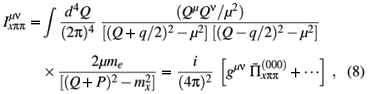where me and mx are the external and the internal baryon masses, respectively. We employ the variables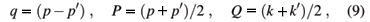where p and p¢ are the initial and final baryon momenta, respectively, whereas k and k¢ are the momenta of the exchanged pions.

The Feynman techniques for loop integration allow one to write the regular part of (8) aswithIn our calculational procedure we use configuration space expressions to obtain sigma-terms. Therefore, we need to perform Fourier transforms in the loop integrals,with x = µr e k = q/µ.

Thus the configuration space equivalent for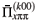can be written as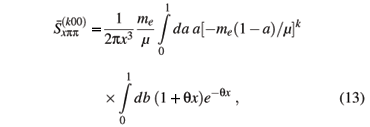whereand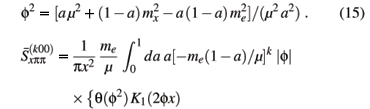The above integral can acquire an imaginary part. This fact becomes evident when we perform the integral in the variable b,In general, when (µ + mx) > me, the function f2 is always positive. On the other hand, when (µ + mx) < me, there is an interval in the integration variable a for which the function f2 becomes negative. In case this happens, the functions Kl must be replaced by Yl. The former are monotonic functions, whereas the latter oscillate and produce contributions with alternating signs inside the integrals. This kind of behaviour is illustrated in Fig. 3, for the arbitrary choices me = 1150 MeV, µ = 150 MeV. In this case the decay threshold corresponds to mx = 1000 MeV.

This is the reason why the contributions in the case (µ + mx) < me, which represents the fact that the external particle can decay, tend to be small. As this is a general feature of the Feynman diagrams, it happens whenever an unstable particle is present. One must bear in mind, however, that the size of this effect depends on both the coupling constants of the specific problem and on the gap me- mx -µ. It is interesting to note that the importance of understanding the smallness of the delta sigma-term has been recently stressed in a conference talk by Meissner  and, to our knowledge, our paper is the only one proposing that the solution to this problem may be associated with the instability of the delta.

IV. SUMMARY

We have reviewed a model aimed at determining sigma-terms, which consists in cutting off configuration space expressions at the point where, as we assume, a phase transition occurs. Our main results are 43 MeV < sN< 49 MeV and 28 MeV < sD < 32 MeV, depending on the coupling constants employed. We have shown that the decay D ® pN changes the behaviour of loop integrals and gives rise to an oscillation in the cloud N contribution to sD which is responsible for the relation sD < sN.

Acknowledgments

This work was supported by CNPq, FAPESP and FUNDECT/MS (Brazilian agencies).

 T. R. Hemmert, B. R. Holstein, and J. Kambor, J. Phys. G 24, 1831 (1998).

 N. Kaiser, S. Gerstendörfer, and W. Weise, Nucl. Phys. A 637, 395 (1998); N. Fettes and Ulf-G. Meissner, Nucl. Phys. A 693, 693 (2001).

 H. Hellmann, Einführung in die Quantenchemie (Deuticke Verlag, Leipzig, 1937); R.P. Feynman, Phys. Rev. 56, 340 (1939); S. T. Epstein, Am. J. Phys. 22, 613 (1954);

 J. Gasser, H. Leutwyler, and M. E. Sainio, Phys. Lett. B 253, 252 (1991); 253, 260 (1991).

 J. Gasser, M.E. Sainio, and A. Svarc, Nucl. Phys. B 307, 779 (1988).

 T. Becher and H. Leutwyler, Eur. Phys. Journal C 9, 643 (1999); JHEP 6, 17 (2001).

 I. P. Cavalcante, M. R. Robilotta, J. Sa Borges, D. de O. Santos, and G. R. S. Zarnauskas, Phys. Rev. C 72, 065207 (2005).

 M. R. Robilotta, Phys. Rev. C 63, 044004 (2001).

 C. Fronsdal, N. Cim. Suppl. 9, 416 (1958).

 V. E. Lyubovitskij, Th. Gutsche, A. Faessler, and E. G. Drukarev, Phys. Rev. D 63, 054026 (2001).

 V. Bernard, T. R. Hemmert, and Ulf-G. Meissner, Phys. Lett. B 622, 141 (2005).

 Ulf-G. Meissner, Proc. Sci. LATT2005, 009 (2005).

•  T. R. Hemmert, B. R. Holstein, and J. Kambor, J. Phys. G 24, 1831 (1998).
•  N. Kaiser, S. Gerstendörfer, and W. Weise, Nucl. Phys. A 637, 395 (1998);
• N. Fettes and Ulf-G. Meissner, Nucl. Phys. A 693, 693 (2001).
•  H. Hellmann, Einführung in die Quantenchemie (Deuticke Verlag, Leipzig, 1937);
• R.P. Feynman, Phys. Rev. 56, 340 (1939);
• S. T. Epstein, Am. J. Phys. 22, 613 (1954);
•  J. Gasser, H. Leutwyler, and M. E. Sainio, Phys. Lett. B 253, 252 (1991);
•  J. Gasser, M.E. Sainio, and A. Svarc, Nucl. Phys. B 307, 779 (1988).
•  T. Becher and H. Leutwyler, Eur. Phys. Journal C 9, 643 (1999);
• JHEP 6, 17 (2001).
•  I. P. Cavalcante, M. R. Robilotta, J. Sa Borges, D. de O. Santos, and G. R. S. Zarnauskas, Phys. Rev. C 72, 065207 (2005).
•  M. R. Robilotta, Phys. Rev. C 63, 044004 (2001).
•  C. Fronsdal, N. Cim. Suppl. 9, 416 (1958).
•  V. E. Lyubovitskij, Th. Gutsche, A. Faessler, and E. G. Drukarev, Phys. Rev. D 63, 054026 (2001).
•  V. Bernard, T. R. Hemmert, and Ulf-G. Meissner, Phys. Lett. B 622, 141 (2005).
•  Ulf-G. Meissner, Proc. Sci. LATT2005, 009 (2005).

# Publication Dates

• Publication in this collection
24 Apr 2007
• Date of issue
Mar 2007